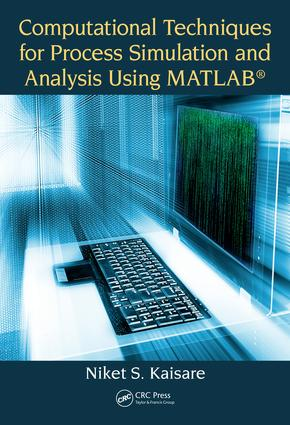Computational Techniques for Process Simulation and Analysis Using MATLAB®

1st Edition

CRC Press

538 pages

Paperback: 9781138746084
pub: 2017-09-19
\$82.95
x
Hardback: 9781498762113
pub: 2017-09-19
\$165.00
x
eBook (VitalSource) : 9781315119519
pub: 2017-09-18
from \$39.98

FREE Standard Shipping!

Description

MATLAB® has become one of the prominent languages used in research and industry and often described as "the language of technical computing". The focus of this book will be to highlight the use of MATLAB® in technical computing; or more specifically, in solving problems in Process Simulations. This book aims to bring a practical approach to expounding theories: both numerical aspects of stability and convergence, as well as linear and nonlinear analysis of systems.

The book is divided into three parts which are laid out with a "Process Analysis" viewpoint. First part covers system dynamics followed by solution of linear and nonlinear equations, including Differential Algebraic Equations (DAE) while the last part covers function approximation and optimization. Intended to be an advanced level textbook for numerical methods, simulation and analysis of process systems and computational programming lab, it covers following key points

• Comprehensive coverage of numerical analyses based on MATLAB for chemical process examples.

• Includes analysis of transient behavior of chemical processes.

• Discusses coding hygiene, process animation and GUI exclusively.

• Treatment of process dynamics, linear stability, nonlinear analysis and function approximation through contemporary examples.

• Focus on simulation using MATLAB to solve ODEs and PDEs that are frequently encountered in process systems.

CHAPTER 1 INTRODUCTION

1.1. A GENERAL MODEL

1.2. STRUCTURE OF A MATLAB CODE

1.3. APPROXIMATIONS AND ERRORS IN NUMERICAL METHODS

1.4. ERROR ANALYSIS

1.5. PROCESS EXAMPLES: A PRELUDE

CHAPTER 2 LINEAR ALGEBRA

2.1. INTRODUCTION

2.2. VECTOR SPACES

2.3. SINGULAR VALUE DECOMPOSITION

2.4. EIGENVALUES AND EIGENVECTORS

2.5. EPILOGUE

2.6. EXERCISES

CHAPTER 3 ORDINARY DIFFERENTIAL EQUATIONS: EXPLICIT METHODS

3.1. GENERAL SETUP

3.2. SECOND ORDER METHODS – A JOURNEY THROUGH THE WOODS

3.3. HIGHER-ORDER RUNGE-KUTTA METHODS

3.4. MATLAB ODE45 SOLVER: OPTIONS AND PARAMETERIZATION

3.5. CASE STUDIES AND EXAMPLES

3.6. EPILOGUE

3.7. EXERCISES

CHAPTER 4 PARTIAL DIFFERENTIAL EQUATIONS IN TIME

4.1. GENERAL SETUP

4.2. A BRIEF OVERVIEW OF NUMERICAL METHODS

4.3. HYPERBOLIC PDE: CONVECTIVE SYSTEMS

4.4. PARABOLIC PDE: DIFFUSIVE SYSTEMS

4.5. CASE STUDIES AND EXAMPLES

4.6. EPILOGUE

4.7. EXERCISES

CHAPTER 5 SECTION WRAP-UP: SIMULATION AND ANALYSIS

5.1. BINARY DISTILLATION COLUMN: STAGED ODE MODEL

5.2. STABILITY ANALYSIS FOR LINEAR SYSTEMS

5.3. COMBINED PARABOLIC PDE WITH ODE-IVP: POLYMER CURING

5.4. TIME VARYING INLET CONDITIONS AND PROCESS DISTURBANCES

5.5. SIMULATING SYSTEM WITH BOUNDARY CONSTRAINTS

5.6. WRAP-UP

5.7. EXERCISES

CHAPTER 6 NONLINEAR ALGEBRAIC EQUATIONS

6.1. GENERAL SETUP

6.2. EQUATIONS IN SINGLE VARIABLE

6.3. NEWTON-RAPHSON: EXTENSIONS AND MULTI-VARIATE

6.4. MATLAB SOLVERS

6.5. CASE STUDIES AND EXAMPLES

6.6. EPILOGUE

6.7. EXERCISES

CHAPTER 7 SPECIAL METHODS FOR LINEAR AND NONLINEAR EQUATIONS

7.1. GENERAL SETUP

7.2. TRI-DIAGONAL AND BANDED SYSTEMS

7.3. ITERATIVE METHODS

7.4. NONLINEAR BANDED SYSTEMS

7.5. EXAMPLES

7.6. EPILOGUE

7.7. EXERCISES

CHAPTER 8 IMPLICIT METHODS: DIFFERENTIAL AND DIFFERENTIAL ALGEBRAIC SYSTEMS

8.1. GENERAL SETUP

8.2. MULTI-STEP METHODS FOR DIFFERENTIAL EQUATIONS

8.3. IMPLICIT SOLUTIONS FOR DIFFERENTIAL EQUATIONS

8.4. DIFFERENTIAL ALGEBRAIC EQUATIONS (DAES)

8.5. CASE STUDIES AND EXAMPLES

8.6. EPILOGUE

8.7. EXERCISES

CHAPTER 9 SECTION WRAP-UP: NONLINEAR ANALYSIS

9.1. NONLINEAR ANALYSIS OF CHEMOSTAT: "TRANSCRITICAL" BIFURCATION

9.2. NON-ISOTHERMAL CSTR: "TURNING-POINT" BIFURCATION

9.3. LIMIT CYCLE OSCILLATIONS

9.4. SIMULATION OF METHANOL SYNTHESIS IN TUBULAR REACTOR

9.5. TRAJECTORY OF A CRICKET BALL

9.6. EXERCISES

CHAPTER 10 REGRESSION AND PARAMETER ESTIMATION

10.1. GENERAL SETUP

10.2. LINEAR LEAST-SQUARES REGRESSION

10.3. REGRESSION IN MULTIPLE VARIABLES

10.4. NONLINEAR ESTIMATION

10.5. CASE STUDIES AND EXAMPLES

10.6. EPILOGUE

10.7. EXERCISES